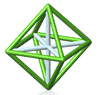Park City Mathematics Institute Secondary School Teachers Program Problem Sets from the Developing Mathematics Course

These course materials are licensed under a Creative Commons Attribution-NonCommercial-ShareAlike 4.0 International License.2019: Developing Mathematical Ideas
Explore connections among seemingly different areas: permutation groups, number theory, and expansions for rational numbers in various bases, all starting from the analysis of perfect card shuffles.2018: Developing Mathematical Ideas
How do we find rational approximations of irrational numbers like pi and sqrt(2)? What happens to the solution sets of equations when we look only for integer solutions? Investigate fractions (of many flavors), greatest common divisors, and irrational numbers in this course.2017: Recursion and Matrices
Explore recursive sequences and develop tools for analyzing them. Develop these tools to take a deep dive into the study of matrices and their many applications.2016: Probability and Big Data
Explore what happens when probability is combined with simple rule-based games. Learn how to calculate expected and long-term game outcomes and why matrix multiplication is defined the way that it is.2015: Some Applications of Geometric Thinking
Exercise some geometric habits of mind like creating functions that can give insight on a geometric problem, or looking for things that don't change when the inputs of a function change.2014: Fractions, tilings, and geometry
Are there any rectangles whose perimeter and area have the same numerical value? Are there any rectangular boxes whose surface area and volume have the same numerical value? Can the plane be tiled with kites and chevrons? In how many ways can 1/2 be written as a sum of unit fractions? How are all of these questions related?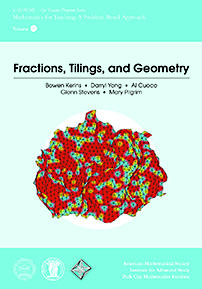Now available: Fractions, Tilings, and Geometry published by American Mathematical Society2013: Probability, Randomization, and Polynomials
Focused on probability, the meaning and definition of standard deviation and variance, the effects of repeating an experiment many times, and ways to generate or test random data.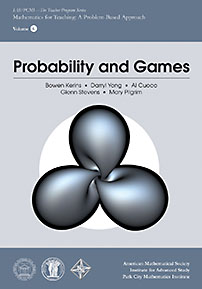Now available: Probability and Games published by American Mathematical Society2012: Moving Things Around: Card Shuffles, Repeating Decimals and Geometric Transformations
Focused on showing how the geometry of polygons is connected to the algebra of the transformations that preserve that geometry.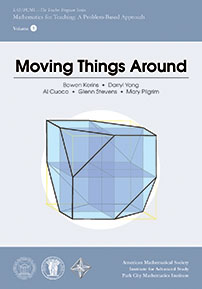Now available: Moving Things Around published by American Mathematical Society2011: Some Applications of Geometric Thinking
Focused on geometry as a useful tool for studying and understanding all kinds of phenomena inside and outside mathematics.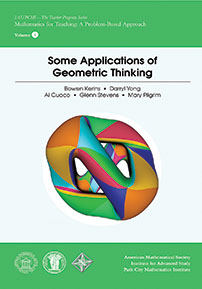Now available: Some Applications of Geometric Thinking published by American Mathematical Society2010: Over and Over
Focused on learning mathematics by working problems together, this course will explore how iterative processes can be used to investigate Fibonacci numbers, image processing, the calculation of square roots, and more.2009: Some Questions and Problems in Arithmetic
Focused on learning mathematics by working problems together, this course explores the algebra of sequences and series as a general-purpose tool for a variety of investigations.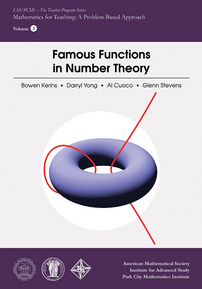Now available: Famous Functions in Number Theory published by American Mathematical Society2008: Applications of Algebra and Geometry to the Craft of Teaching
Focused on learning mathematics by working problems together, this course will use this theme as a springboard into investigations of the structure of different algebraic systems and geometric curves. This applied mathematics - choosing and designing tasks - is mathematics applied to the work teachers do.Now available: Applications of Algebra and Geometry to the Work of Teaching published by American Mathematical Society2007: Developing Mathematics: Probability Through Algebra
Focused on learning mathematics by working problems together, this course explores the fundamental mathematics on a topic that has its roots in secondary level, and is related to the mathematical theme of the Institute.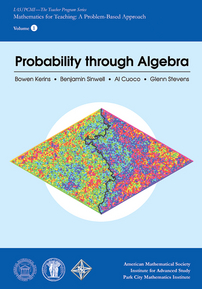Now available: Probability through Algebra published by American Mathematical Society2006: Some Applications of Geometric Thinking
Teachers in this course will look at some basic geometric habits of mind like studying continuous change and looking for things that don't change, and they'll apply these habits to a wide variety of situations.2005: Doing It with Differences
Focused on learning mathematics by working problems together, this course explores the fundamental mathematics on a topic that has its roots in the secondary level, and is related to the mathematical theme of the Institute.2004: Stories that Count
This course looks at how combinatorics itself can fit into the 5-12 program, but it also looks at how combinatorics and combinatorial thinking can be used to illuminate ideas from more mainstream courses like algebra, arithmetic, and geometry.2003: Sums and Differences
This course looks at the calculus of finite differences as a unifying theme for these questions and others that connect to the middle and high school curriculum.2002: Gaussian Integers
Focused exclusively on learning mathematics by working problems together, this course explores the fundamental mathematics on a topic that is rooted at the secondary level but related to the mathematical theme of the Institute.2001: The Euclidean Algorithm
Focused exclusively on learning mathematics by working problems together, this course explores the fundamental algorithm on which much of arithmetic and algebra is based.

Unless otherwise indicated all of these materials are licensed under a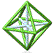© 2001 - 2020 Park City Mathematics Institute IAS/Park City Mathematics Institute is an outreach program of the Institute for Advanced Study, 1 Einstein Drive, Princeton, NJ 08540. Send questions or comments to: Suzanne Alejandre